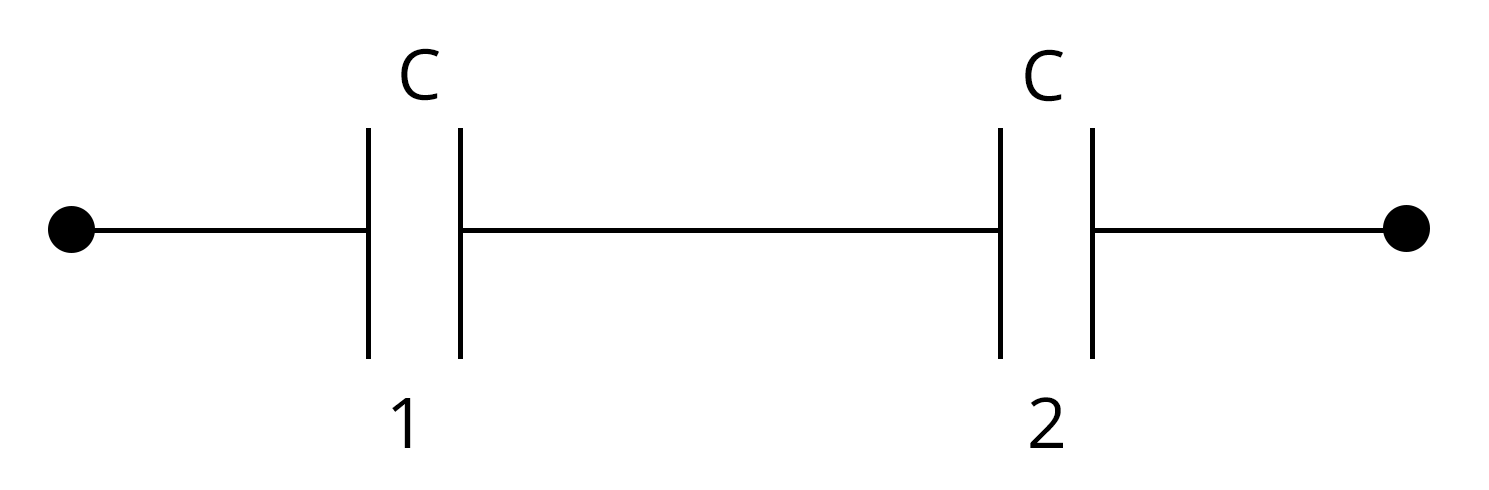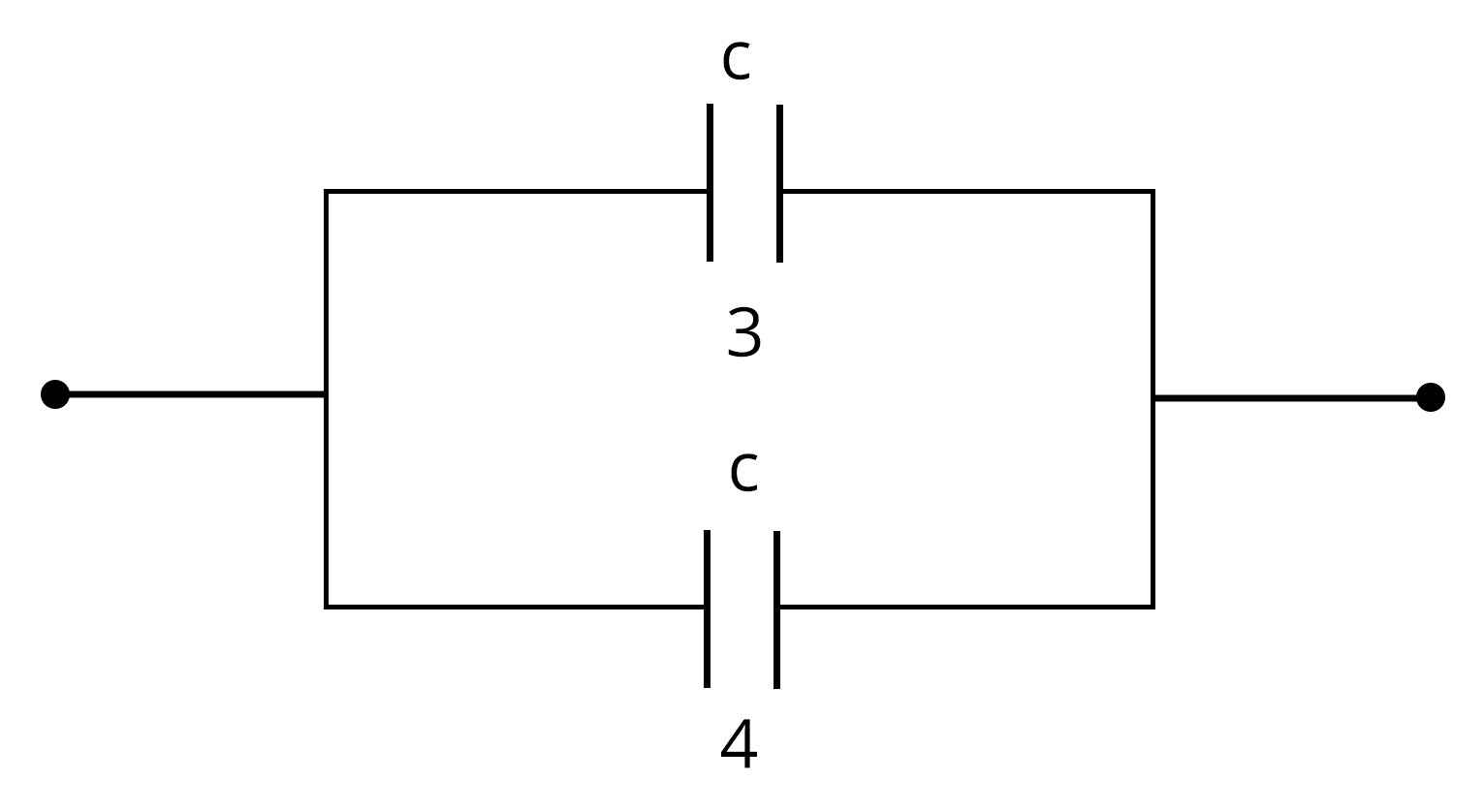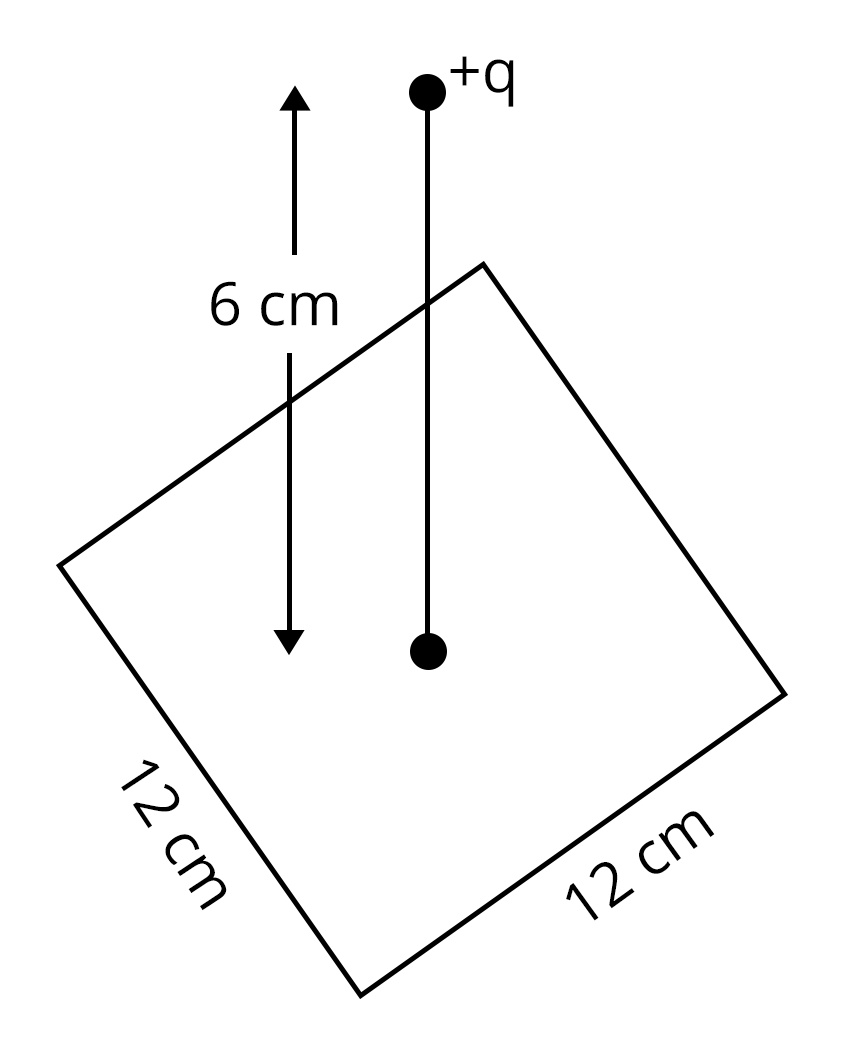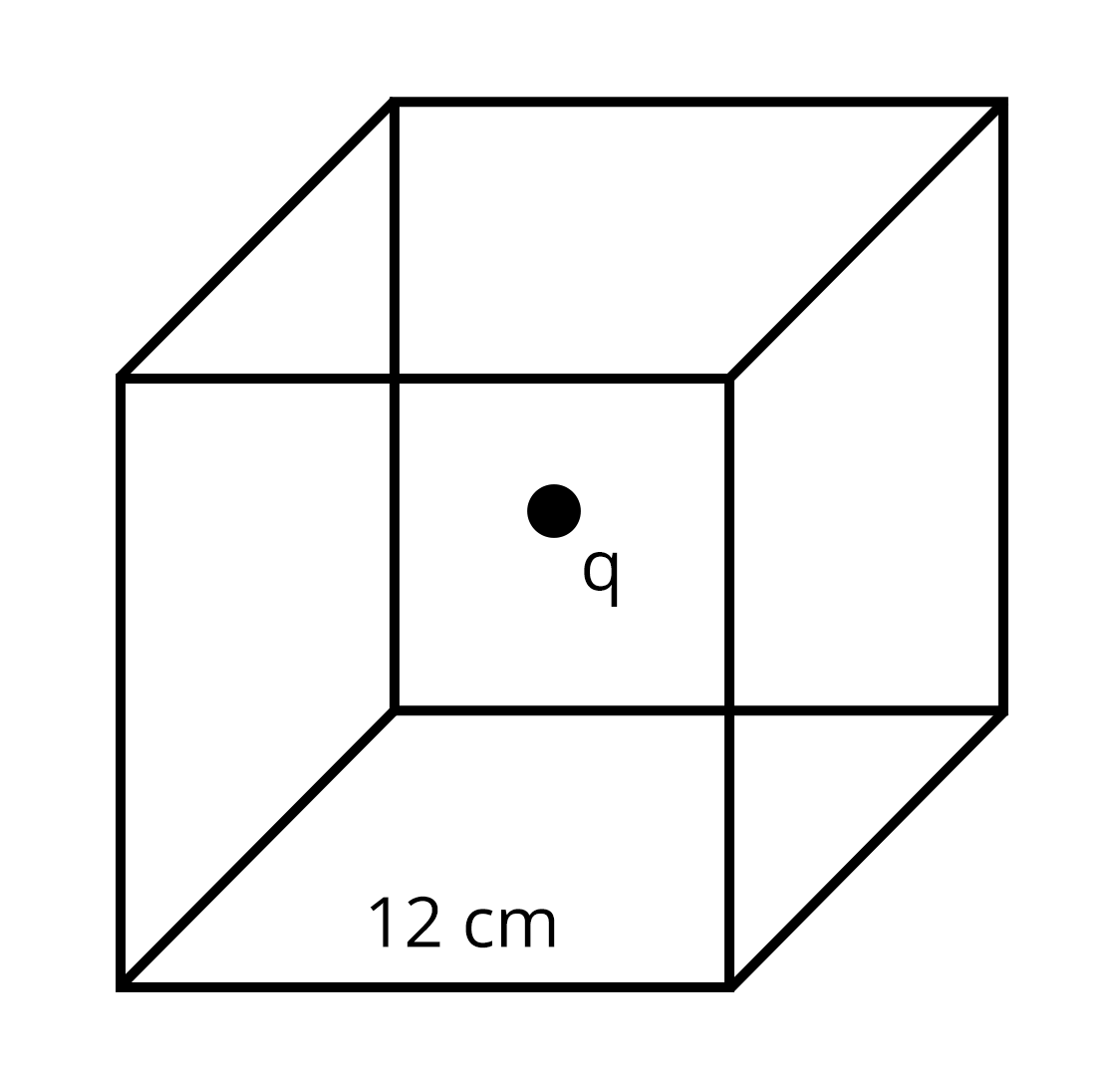Courses
Courses for Kids
Free study material
Free LIVE classes
More

# JEE Important Chapter - Electrostatics

Get interactive courses taught by top teachers## Electrostatics

Last updated date: 20th Sep 2023
Total views: 147.9k
Views today: 5.83k

Electrostatics is the branch of physics that deals with the study of charges at rest and their interaction with other charges. This section consists of concepts and advanced problems related to electrostatics. It is a very important chapter for JEE in terms of weightage.

The chapter Electrostatics begins by introducing what is electrostatics, what is electrostatic charge, Coulomb’s law of electrostatics and then problems related to Coulomb's law of electrostatics. Then, we will see the electric potential and electric field due to point charges and continuous charge distribution of different shapes such as rings, discs, cylinders etc. We can see many examples of electrostatic effects in our life.

The torque experienced by an electric dipole and its potential energy is discussed in this chapter along with applications of Gauss law to calculate the net electric flux linked with a closed surface. Frequent questions are asked for JEE about types of capacitors and problems related to capacitors in series and parallel combinations. The effect of the dielectric medium in a capacitor is also discussed in this section.

Let us see what electrostatics is and define electrostatic force. We will also cover the important formulas needed for JEE Main and JEE Advanced related to Electrostatics with solved examples.

## JEE Main Physics Chapter-wise Solutions 2022-23

### Important Topics in Electrostatics

• Coulomb’s Law.

• Electric field and electric lines of force.

• Electric field due to continuous charge distribution.

• Electric dipole and dipole moment.

• Gauss Law.

• Electric potential and Equipotential surface.

• Electric potential due to the various charge distributions.

• Capacitance of a capacitor.

• Grouping of Capacitance.

### Solved Examples

1. The electric potential in a region is given by V=(6x-8xy2-8y+6yz-4x2) volt. Then the electric field acting on a point charge of 2C placed at the origin will be …

Ans: Given,

The electric potential in a region , V=(6x-8xy2-8y+6yz-4x2) volt

The electric field in the region can be obtained from the electric potential using the formula

$\vec E=\dfrac{\partial V}{\partial x} \hat i+\dfrac{\partial V}{\partial y} \hat j+\dfrac{\partial V}{\partial z} \hat k$

$\vec E=(6-8y^2-8x) \hat i+(-16xy-8+6z) \hat j+6y \hat k$...(1)

To find the electric field at origin, put x=0, y=0 and z=0 in equation (1)

$\vec E=6 \hat i+-8 \hat j+0 \hat k$

$|\vec E|=\sqrt{6^2+(-8)^2}$

$|\vec E|=10~N/C$

The force acting on the charge q due to electric E is given by

$F=qE$

$F=2C\times 10~N/C=20~N$

Key point: Electric field at a point can be obtained by the differentiation of electric potential with respect to distance.

2. A parallel plate capacitor with oil between plates (dielectric constant of oil k=2) has a capacitance C. If the oil is removed, then the capacitance of the capacitor becomes…

Ans: Let A be the area of the capacitor and d be the distance between the plates of the capacitor.

The formula for the  capacitance of the parallel plate capacitor with oil as a dielectric is given  by,

$C=\dfrac{kA\epsilon_0}{d}$...(1)

The capacitance of the parallel plate capacitor when oil is removed is

$C'=\dfrac{A\epsilon_0}{d}$...(2)

Divide equation (2) by (1) to obtain the new capacitance C’ when oil is removed in terms of initial capacitance C.

$\dfrac{C'}{C}=\dfrac{\left(\dfrac{A\epsilon_0}{d}\right)}{\left(\dfrac{kA\epsilon_0}{d}\right)}$

$\dfrac{C'}{C}=\dfrac{1}{k}$

$C'=\dfrac{C}{k}$

When the oil is removed from the parallel plate capacitor, its capacitance is reduced by a factor of k.

Key point:  The capacitance of a parallel plate capacitor depends on the dielectric medium and the dimensions of the capacitor. It does not depend on the charge and potential difference across the capacitor.

### Previous Year Questions from JEE Paper

1. Two equal capacitors are first connected in series and then in parallel. The ratio of equivalent capacitances in the two cases will be (JEE 2021)

Sol:

Let the capacitances of each capacitor be C.

In the series connection of two capacitors, the equivalent capacitances is given by$\dfrac{1}{C_{eq}}=\dfrac{1}{C_1}+\dfrac{1}{C_2}$

$\dfrac{1}{C_{12}}=\dfrac{1}{C}+\dfrac{1}{C}$

$C_{12}=\dfrac{C}{2}$...(1)

For the capacitors in parallel connection, the equivalent capacitance is given by$C_{eq}=C_{3}+C_{4}$

$C_{34}=C+C$

$C_{34}=2C$...(2)

The ratio of  the equivalent capacitances in series and the parallel connection is obtained by dividing equation (1) and equation (2).

$\dfrac{C_{12}}{C_{34}}=\dfrac{\left(\dfrac{C}{2}\right)}{2C}$

$\dfrac{C_{12}}{C_{34}}=\dfrac{1}{4}$

The ratio of the equivalent capacitances of series and parallel connection is 1:4

Key point:  In series connection, equivalent capacitance is equal to the reciprocal of the sum of the reciprocal of each capacitance. For parallel connection, equivalent capacitance is equal to the sum of each individual capacitance.

2. A point charge of +12 μC  is at a distance 6 cm vertically above the centre of a square of side 12 cm as shown in the figure. The magnitude of electric flux through the square will be _____ ✕ 103 Nm2/C. (JEE 2021 Feb)Sol. To find the electric flux, we use Gauss law and assume a Gaussian surface in the form of a cube of side length 12 cm in such a way that charge is located at the centre of the cube.Applying Gauss law, total flux linked with the cube is given by

$\phi_{total}=\dfrac{q}{\epsilon_0}$

The electric flux(ɸ) through the square we want to find is 1/6 times the total flux.

$\phi=\dfrac{q}{6\epsilon_0}$

$\phi=\dfrac{12\times10^{-6}}{6\times8.85\times10^{-12}}$

$\phi=226\times10^{3}~Nm^2/C$

Therefore, the flux through the square will be 226 × 103 Nm2/C.

Key point: Gauss Law is applicable to any Gaussian surface irrespective of size and shape.

### Practice Questions

1. There is 10 mC of charge at the centre of a circle of radius 10 cm. The work done in moving a charge of 1mC around the circle once is

2. A parallel plate capacitor having a plate separation of 2 mm is charged by connecting it to a 300 V supply. The energy density is

Ans: 0.1 J/m3

### Conclusion

In this article, we define electrostatic force, related definitions and formulae. We also solved some JEE numeral problems to understand the types of questions asked in the exam.  You should memorise all the formulae to solve numerical problems. Numerical problems will help you to score good marks in JEE exams.

## Important Related Links for JEE Main 2022-23

See More## JEE Main Important Dates

View all JEE Main Exam Dates
JEE Main 2023 January and April Session exam dates and revised schedule have been announced by the NTA. JEE Main 2023 January and April Session will now be conducted on 24-Jan-2023 to 31-Jan-2023 and 6-Apr-2023 to 12-Apr-2023, and the exam registration closes on 12-Jan-2023 and Apr-2023. You can check the complete schedule on our site. Furthermore, you can check JEE Main 2023 dates for application, admit card, exam, answer key, result, counselling, etc along with other relevant information.
See More
View all JEE Main Exam Dates## JEE Main Information

Application Form
Eligibility Criteria
Reservation Policy
Exam Centres
NTA has announced the JEE Main 2023 January session application form release date on the official website https://jeemain.nta.nic.in/. JEE Main 2023 January and April session Application Form is available on the official website for online registration. Besides JEE Main 2023 January and April session application form release date, learn about the application process, steps to fill the form, how to submit, exam date sheet etc online. Check our website for more details. April Session's details will be updated soon by NTA.## JEE Main Syllabus

View JEE Main Syllabus in Detail
It is crucial for the the engineering aspirants to know and download the JEE Main 2023 syllabus PDF for Maths, Physics and Chemistry. Check JEE Main 2023 syllabus here along with the best books and strategies to prepare for the entrance exam. Download the JEE Main 2023 syllabus consolidated as per the latest NTA guidelines from Vedantu for free.
See More
View JEE Main Syllabus in Detail## JEE Main 2023 Study Material

View all study material for JEE Main
JEE Main 2023 Study Materials: Strengthen your fundamentals with exhaustive JEE Main Study Materials. It covers the entire JEE Main syllabus, DPP, PYP with ample objective and subjective solved problems. Free download of JEE Main study material for Physics, Chemistry and Maths are available on our website so that students can gear up their preparation for JEE Main exam 2023 with Vedantu right on time.
See More
All
Mathematics
Physics
Chemistry
See All## JEE Main Question Papers

see all
Download JEE Main Question Papers & ​Answer Keys of 2022, 2021, 2020, 2019, 2018 and 2017 PDFs. JEE Main Question Paper are provided language-wise along with their answer keys. We also offer JEE Main Sample Question Papers with Answer Keys for Physics, Chemistry and Maths solved by our expert teachers on Vedantu. Downloading the JEE Main Sample Question Papers with solutions will help the engineering aspirants to score high marks in the JEE Main examinations.
See MoreView all JEE Main Important Books
In order to prepare for JEE Main 2023, candidates should know the list of important books i.e. RD Sharma Solutions, NCERT Solutions, RS Aggarwal Solutions, HC Verma books and RS Aggarwal Solutions. They will find the high quality readymade solutions of these books on Vedantu. These books will help them in order to prepare well for the JEE Main 2023 exam so that they can grab the top rank in the all India entrance exam.
See More
Maths
NCERT Book for Class 12 Maths
Physics
NCERT Book for Class 12 Physics
Chemistry
NCERT Book for Class 12 Chemistry
Physics
H. C. Verma Solutions
Maths
R. D. Sharma Solutions
Maths
R.S. Aggarwal Solutions
See All## JEE Main Mock Tests

View all mock tests
JEE Main 2023 free online mock test series for exam preparation are available on the Vedantu website for free download. Practising these mock test papers of Physics, Chemistry and Maths prepared by expert teachers at Vedantu will help you to boost your confidence to face the JEE Main 2023 examination without any worries. The JEE Main test series for Physics, Chemistry and Maths that is based on the latest syllabus of JEE Main and also the Previous Year Question Papers.
See More## JEE Main 2023 Cut-Off

JEE Main Cut Off
NTA is responsible for the release of the JEE Main 2023 January and April Session cut off score. The qualifying percentile score might remain the same for different categories. According to the latest trends, the expected cut off mark for JEE Main 2023 January and April Session is 50% for general category candidates, 45% for physically challenged candidates, and 40% for candidates from reserved categories. For the general category, JEE Main qualifying marks for 2021 ranged from 87.8992241 for general-category, while for OBC/SC/ST categories, they ranged from 68.0234447 for OBC, 46.8825338 for SC and 34.6728999 for ST category.
See More## JEE Main 2023 Results

NTA will release the JEE Main 2023 January and April sessions exam dates on the official website, i.e. {official-website}. Candidates can directly check the date sheet on the official website or https://jeemain.nta.nic.in/. JEE Main 2023 January and April sessions is expected to be held in February and May. Visit our website to keep updates of the respective important events of the national entrance exam.
See More
Rank List
Counselling
Cutoff
JEE Main 2023 state rank lists will be released by the state counselling committees for admissions to the 85% state quota and to all seats in IIT colleges. JEE Main 2023 state rank lists are based on the marks obtained in entrance exams. Candidates can check the JEE Main 2023 state rank list on the official website or on our site.## JEE Top Colleges

View all JEE Main 2023 Top Colleges
Want to know which Engineering colleges in India accept the JEE Main 2023 scores for admission to Engineering? Find the list of Engineering colleges accepting JEE Main scores in India, compiled by Vedantu. There are 1622 Colleges that are accepting JEE Main. Also find more details on Fees, Ranking, Admission, and Placement.
See More## FAQs on JEE Important Chapter - Electrostatics

FAQ

1. What is the weightage of the electrostatics in JEE?

Electrostatics is a vast chapter compared to others. Around 2-3 questions covering about 8-12 marks are asked in JEE from various parts of the chapter.

2. Which section has to be given more importance in the chapter on electrostatics?

Since electrostatic is a vast topic, there are many important concepts here in this chapter. However, more focus should be given to the electric fields of various charge distribution, Gauss law and capacitance to score better marks in JEE

3. How to score good marks in JEE from the chapter electrostatics?

If we go through the last 20 years’ question papers, we can understand the important concepts of the chapter from where the questions are asked frequently. Understanding these concepts in-depth and then solving practice problems is the best way to score good marks in JEE for the chapter electrostatics.## Notice board

JEE News
JEE Blogs